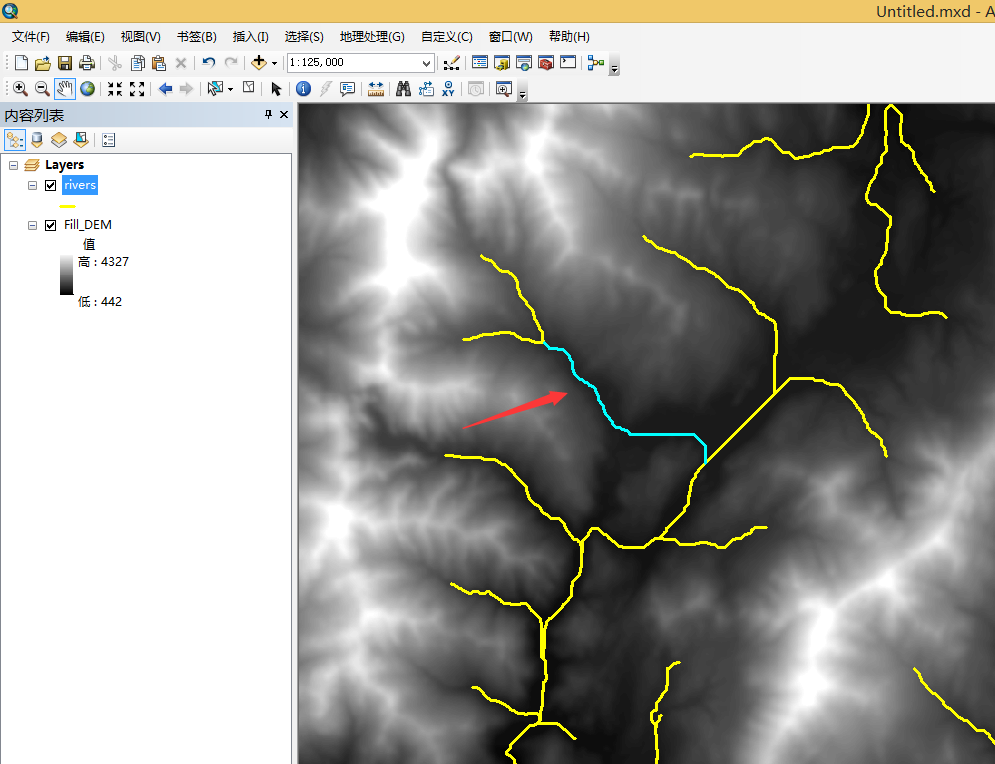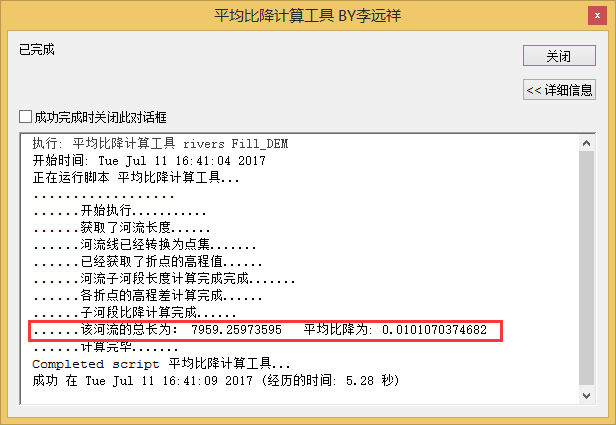# ArcGIS水文分析实战教程（10）河流平均比降计算

### 河流比降S—- 河段平均河底比降
Li—-第i个子河段长度
Si—-第i个子河段河底比降
N—- 子河段数量

### 河流比降与GIS的关系

1. 如果河流数据与DEM数据不是同一份数据，那么像元点与河流线的重合程度有待商榷。

2. 如果河流数据与DEM数据吻合（或者河流数据直接从DEM数据提取出来的），其高程的获取所依赖的DEM必须是填洼后的无凹陷点DEM。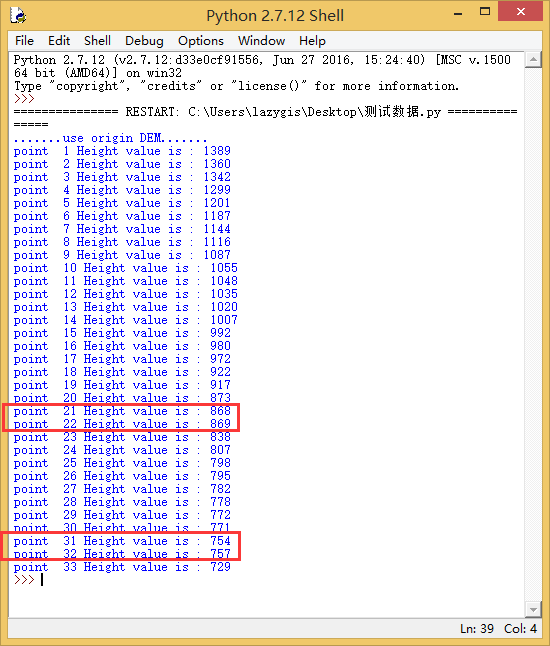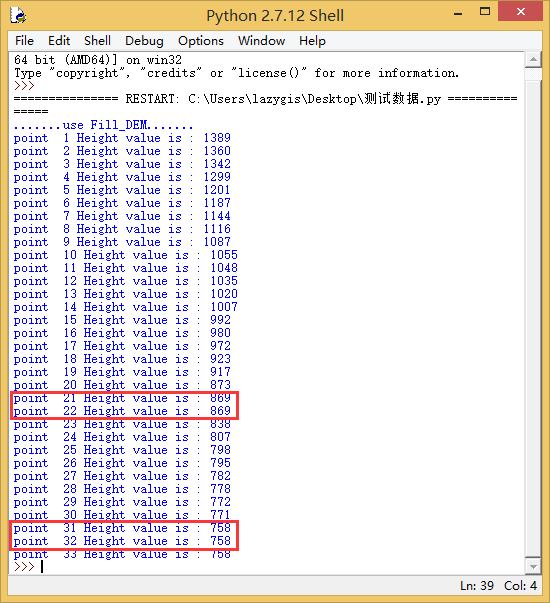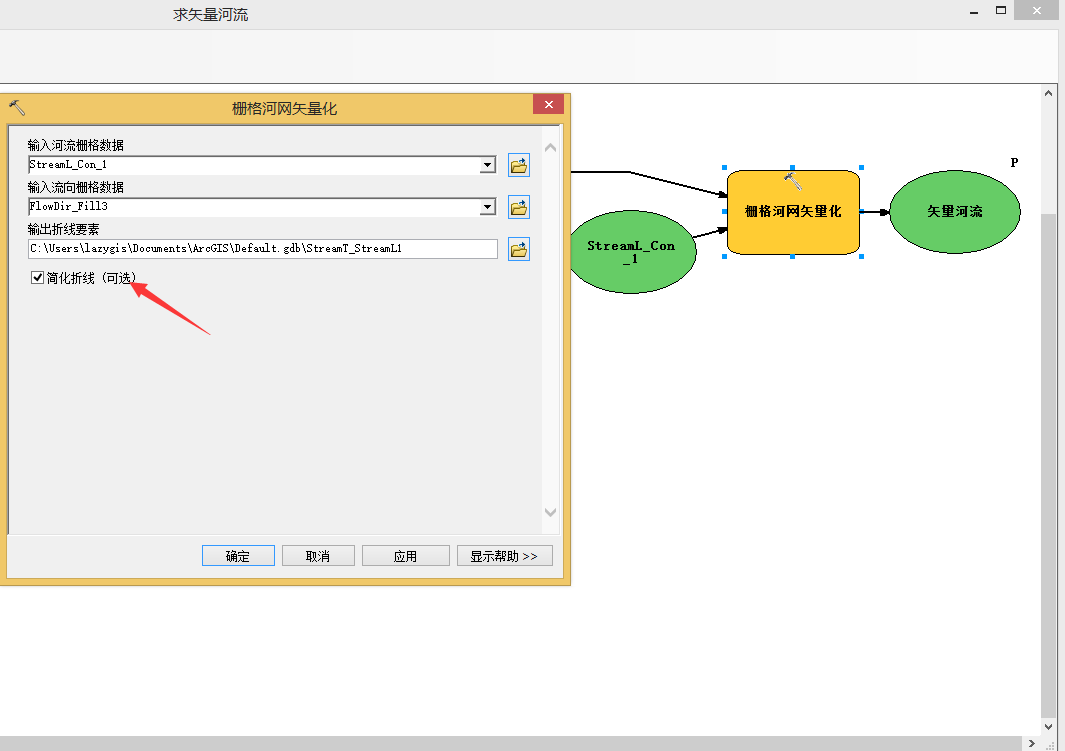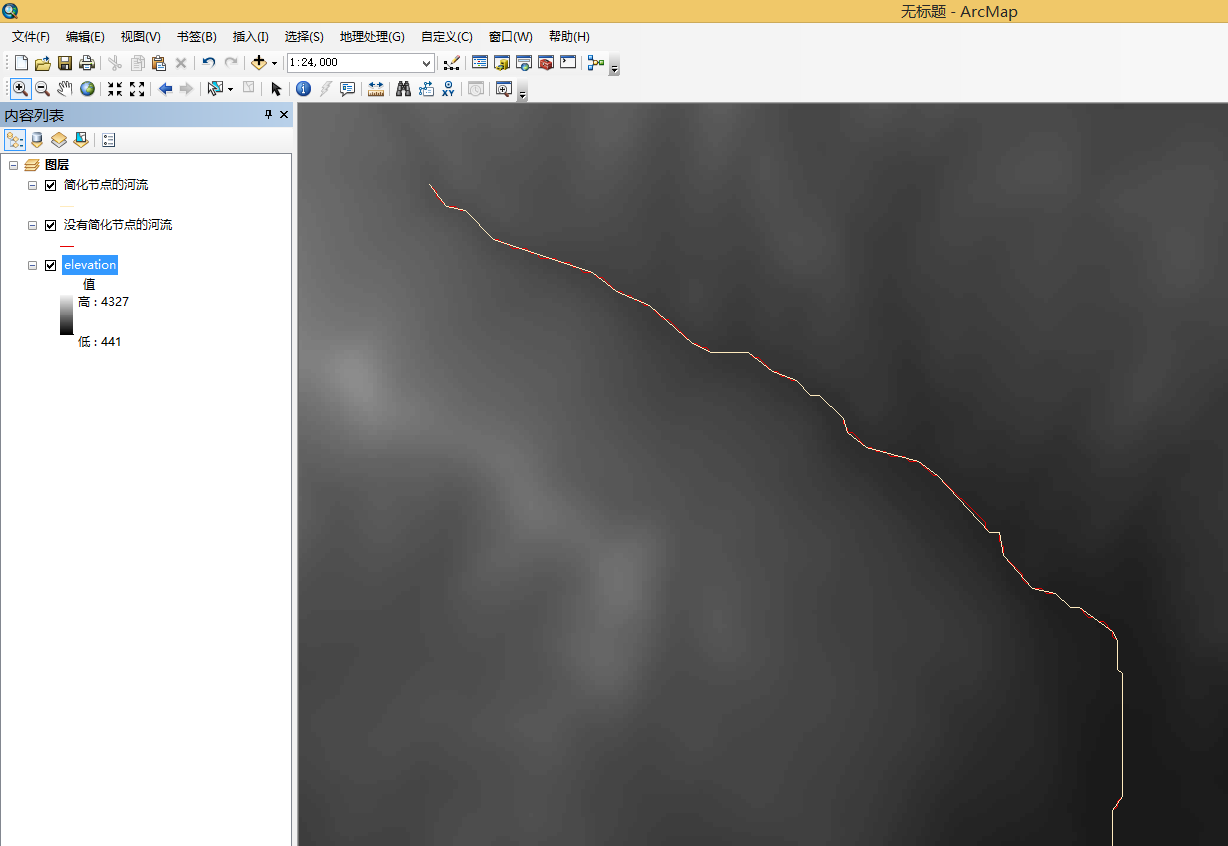### ArcGIS中实现河流平均比降计算

ArcGIS 除了前面章节提及到的水文分析工具及其相关工具之外，还有非常庞大的工具集对空间计算进行支撑。由于没有断面数据的支撑，这里只考虑通过地形和河流线型进行计算，也就是说实现【约翰斯通-克罗斯】法。

1. 通过河流线提取河流折点，利用这些折点与DEM数据叠加，获取高程值

2. 利用河流折点，获取其坐标值，根据两点距离公式，计算每一段子河段的长度

3. 根据每个点的高程值，计算出相邻两点之间的高差

4. 根据高差与子河段长度，计算出各子河段的河底比降

5. 汇总这些数据计算出整个河段的平均河底比降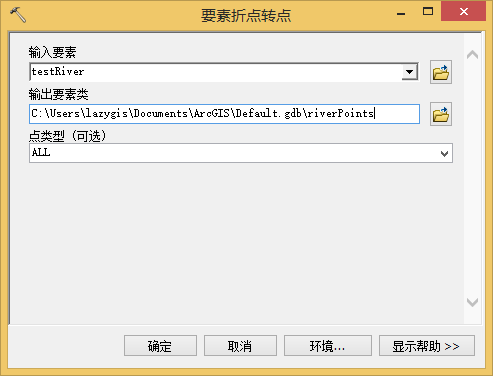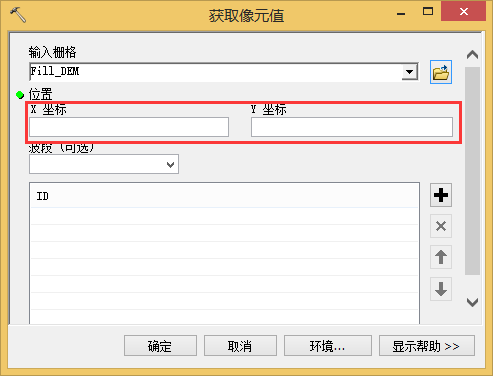``````    # 获取河流的节点，以用作计算高差和河长
for row in arcpy.da.SearchCursor(outLine1, ["SHAPE@XY"]):
# 从点图层数据获取所有的点的XY
x, y = row
#从地形数据中获取其高程的象元值
pointStr = str(x) +" "+str(y)
result = arcpy.GetCellValue_management(dem,pointStr)
riverPoints.append(row)
heightList.append(str(result))
``````

``````#############################################################
#                                                           #
#   该工具利用【约翰斯通-克罗斯】方法计算河底平均比降           #
#   在使用的时候必须先选择一条河流，工具会判断输入的河流数量     #
#   大于一条河流时不予以计算                                  #
#   用于计算的DEM为无凹陷点DEM                                #
#   BY 李远祥                                                #
#                                                            #
##############################################################

# -*- coding: gbk -*-
import arcpy
import math
inputLine =arcpy.GetParameterAsText(0) #河流图层

dem = arcpy.GetParameterAsText(1) #无凹陷点DEM
riverLen = 7873.232038

# 记录高程值的数据
heightList = []

iputCout=0
mmCursor = arcpy.da.SearchCursor(inputLine, ["SHAPE@LENGTH"])
for row1 in mmCursor:
riverLen = row1      #顺便获取河流长度
iputCout=iputCout+1

#如果选中的是一个线要素，则执行计算，否者不计算多条河流
if iputCout==1:
##先将线转为折点
outLine1 = arcpy.FeatureVerticesToPoints_management(inputLine, "in_memory/outline")

#河流节点
riverPoints = []

# 获取河流的节点，以用作计算高差和河长
for row in arcpy.da.SearchCursor(outLine1, ["SHAPE@XY"]):
# 从点图层数据获取所有的点的XY
x, y = row
#从地形数据中获取其高程的象元值
pointStr = str(x) +" "+str(y)
result = arcpy.GetCellValue_management(dem,pointStr)
riverPoints.append(row)
heightList.append(str(result))

#子河段长度

subRiverList = []
hDiffList = []
# 计算每一个子河段的长度
for i in range(0,len(riverPoints)-1):
x,y = riverPoints[i]
x1,y1 = riverPoints[i+1]
#计算河段长度
dis =math.sqrt(pow((x1-x),2)+pow((y1-y),2))
subRiverList.append(dis)

# 计算从高程从低到高部分的差距
listLen = len(heightList)
for j in range(0,listLen-1):
h1 = float(heightList[j])
h2 = float(heightList[j+1])
xheight = 0
xheight = h1-h2
hDiffList.append(xheight)

# 计算每个河段的比降
subbijiang = []
for i in range(len(hDiffList)):
Si= hDiffList[i]/subRiverList[i]
subbijiang.append(Si)

# 计算最终的比降
# 计算分子
sumUp = 0
for i in range(len(hDiffList)-1):
sumUp = sumUp + subRiverList[i]*pow(subbijiang[i],0.5)

S = pow((sumUp/riverLen),2)

arcpy.AddMessage(u'......该河流的总长为： ' + str(riverLen)+u'   平均比降为: ' +str(S))

else:

``````

### 河流平均比降工具使用